## Electric vs. Magnetic Forces

Electric and magnetic forces both affect the trajectory of charged particles, but in qualitatively different ways.

### Learning Objectives

Compare the effects of the electric and the magnetic fields on the charged particle

### Key Takeaways

#### Key Points

• The force on a charged particle due to an electric field is directed parallel to the electric field vector in the case of a positive charge, and anti-parallel in the case of a negative charge. It does not depend on the velocity of the particle.
• In contrast, the magnetic force on a charge particle is orthogonal to the magnetic field vector, and depends on the velocity of the particle. The right hand rule can be used to determine the direction of the force.
• An electric field may do work on a charged particle, while a magnetic field does no work.
• The Lorentz force is the combination of the electric and magnetic force, which are often considered together for practical applications.
• Electric field lines are generated on positive charges and terminate on negative ones. The field lines of an isolated charge are directly radially outward. The electric field is tangent to these lines.
• Magnetic field lines, in the case of a magnet, are generated at the north pole and terminate on a south pole. Magnetic poles do not exist in isolation. Like in the case of electric field lines, the magnetic field is tangent to the field lines. Charged particles will spiral around these field lines.

#### Key Terms

• orthogonal: Of two objects, at right angles; perpendicular to each other.

### Electric vs. Magnetic Forces

Force due to both electric and magnetic forces will influence the motion of charged particles. However, the resulting change to the trajectory of the particles will differ qualitatively between the two forces. Below we will quickly review the two types of force and compare and contrast their effects on a charged particle.

### Electrostatic Force and Magnetic Force on a Charged Particle

Recall that in a static, unchanging electric field E the force on a particle with charge q will be:

$\text{F}=\text{qE}$

Where F is the force vector, q is the charge, and E is the electric field vector. Note that the direction of F is identical to E in the case of a positivist charge q, and in the opposite direction in the case of a negatively charged particle. This electric field may be established by a larger charge, Q, acting on the smaller charge q over a distance r so that:

$\text{E}=\left | \frac{\text{F}}{\text{q}} \right |=\text{k} \left | \frac{\text{qQ}}{\text{qr}^{2}} \right |=\text{k}\frac{\left | \text{Q} \right |}{\text{r}^{2}}$

It should be emphasized that the electric force F acts parallel to the electric field E. The curl of the electric force is zero, i.e.:

$\bigtriangledown \times \text{E}=0$

A consequence of this is that the electric field may do work and a charge in a pure electric field will follow the tangent of an electric field line.

In contrast, recall that the magnetic force on a charged particle is orthogonal to the magnetic field such that:

$\text{F}=\text{qv} \times \text{B}=\text{qvBsin}\theta$

where B is the magnetic field vector, v is the velocity of the particle and θ is the angle between the magnetic field and the particle velocity. The direction of F can be easily determined by the use of the right hand rule.Right Hand Rule: Magnetic fields exert forces on moving charges. This force is one of the most basic known. The direction of the magnetic force on a moving charge is perpendicular to the plane formed by v and B and follows right hand rule–1 (RHR-1) as shown. The magnitude of the force is proportional to q, v, B, and the sine of the angle between v and B.

If the particle velocity happens to be aligned parallel to the magnetic field, or is zero, the magnetic force will be zero. This differs from the case of an electric field, where the particle velocity has no bearing, on any given instant, on the magnitude or direction of the electric force.

The angle dependence of the magnetic field also causes charged particles to move perpendicular to the magnetic field lines in a circular or helical fashion, while a particle in an electric field will move in a straight line along an electric field line.

A further difference between magnetic and electric forces is that magnetic fields do not net work, since the particle motion is circular and therefore ends up in the same place. We express this mathematically as:

$\text{W}=\oint \text{B} \cdot \text{dr} = 0$

### Lorentz Force

The Lorentz force is the combined force on a charged particle due both electric and magnetic fields, which are often considered together for practical applications. If a particle of charge q moves with velocity v in the presence of an electric field E and a magnetic field B, then it will experience a force:

$\text{F}=\text{q}[\text{E}+\text{vBsin}\theta]$

### Electric and Magnetic Field Lines

We mentioned briefly above that the motion of charged particles relative to the field lines differs depending on whether one is dealing with electric or magnetic fields. There are some notable differences between how electric and magnetic field lines are conceptualized. The electric field lines from a positive isolated charge are simply a sequence of evenly-spaced, radially directed lines pointed outwards from the charge. In the case of a negative charge, the direction of the field is reversed. The electric field is directed tangent to the field lines. Of course, we imagine the field lines are more densely packed the larger the charges are. One can see clearly that the curl of the electric force is zero.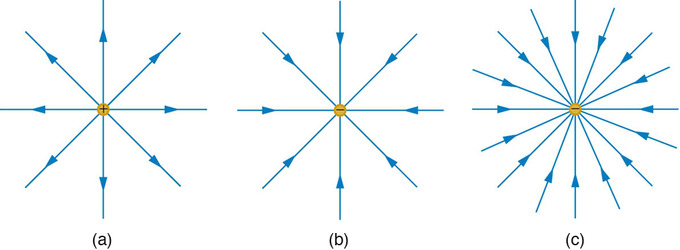Electric Field Generated by Point Charges: The electric field surrounding three different point charges: (a) A positive charge; (b) a negative charge of equal magnitude; (c) a larger negative charge.

If multiple charges are involved, field lines are generated on positive charges, and terminate on negative ones.

In the case of magnets, field lines are generated on the north pole (+) and terminate on the south pole (-) – see the below figure. Magnetic ‘charges’, however, always come in pairs – there are no magnetic monopoles (isolated north or south poles). The curl of a magnetic field generated by a conventional magnet is therefore always non zero. Charged particles will spiral around these field lines, as long as the particles have some non-zero component of velocity directed perpendicular to the field lines.Magnetic Pole Model: The magnetic pole model: two opposing poles, North (+) and South (−), separated by a distance d produce an H-field (lines).

A magnetic field may also be generated by a current with the field lines envisioned as concentric circles around the current-carrying wire.The magnetic force at any point in this case can be determined with the right hand rule, and will be perpendicular to both the current and the magnetic field.

## Constant Velocity Produces a Straight-Line

If a charged particle’s velocity is parallel to the magnetic field, there is no net force and the particle moves in a straight line.

### Learning Objectives

Identify conditions required for the particle to move in a straight line in the magnetic field

### Key Takeaways

#### Key Points

• Newton’s first law of motion states that if an object experiences no net force, then its velocity is constant.
• A particle with constant velocity will move along a straight line through space.
• If a charged particle’s velocity is completely parallel to the magnetic field, the magnetic field will exert no force on the particle and thus the velocity will remain constant.
• In the case that the velocity vector is neither parallel nor perpendicular to the magnetic field, the component of the velocity parallel to the field will remain constant.

#### Key Terms

• straight-line motion: motion that proceeds in a single direction

### Constant Velocity Produces Straight-Line Motion

Recall Newton’s first law of motion. If an object experiences no net force, then its velocity is constant: the object is either at rest (if its velocity is zero), or it moves in a straight line with constant speed (if its velocity is nonzero).

There are many cases where a particle may experience no net force. The particle could exist in a vacuum far away from any massive bodies (that exert gravitational forces) and electromagnetic fields. Or there could be two or more forces on the particle that are balanced such that the net force is zero. This is the case for, say, a particle suspended in an electric field with the electric force exactly counterbalancing gravity.

If the net force on a particle is zero, then the acceleration is necessarily zero from Newton’s second law: F=ma. If the acceleration is zero, any velocity the particle has will be maintained indefinitely (or until such time as the net force is no longer zero). Because velocity is a vector, the direction remains unchanged along with the speed, so the particle continues in a single direction, such as with a straight line.

### Charged Particles Moving Parallel to Magnetic Fields

The force a charged particle “feels” due to a magnetic field is dependent on the angle between the velocity vector and the magnetic field vector B . Recall that the magnetic force is: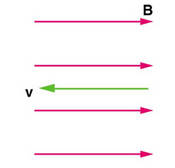Zero Force When Velocity is Parallel to Magnetic Field: In the case above the magnetic force is zero because the velocity is parallel to the magnetic field lines.

$\text{F}=\text{qvBsin} \theta$

If the magnetic field and the velocity are parallel (or antiparallel), then sinθ equals zero and there is no force. In this case a charged particle can continue with straight-line motion even in a strong magnetic field. If is between 0 and 90 degrees, then the component of v parallel to B remains unchanged.

## Circular Motion

Since the magnetic force is always perpendicular to the velocity of a charged particle, the particle will undergo circular motion.

### Learning Objectives

Describe conditions that lead to the circular motion of a charged particle in the magnetic field

### Key Takeaways

#### Key Points

• The magnetic field does no work, so the kinetic energy and speed of a charged particle in a magnetic field remain constant.
• The magnetic force, acting perpendicular to the velocity of the particle, will cause circular motion.
• The centripetal force of the particle is provided by magnetic Lorentzian force so that $\text{qvB}=\frac{\text{mv}^{2}}{\text{r}}$.
• Solving for r above yields the gryoradius, or the radius of curvature of the path of a particle with charge q and mass m moving in a magnetic field of strength B. The gryoradius is then given by $\text{r}=\frac{\text{mv}}{\text{qB}}$.
• The cyclotron frequency (or, equivalently, gyrofrequency) is the number of cycles a particle completes around its circular circuit every second and is given by $\text{f}=\frac{\text{qB}}{2 \pi \text{m}}$.

#### Key Terms

• gyroradius: The radius of the circular motion of a charged particle in the presence of a uniform magnetic field.
• cyclotron frequency: The frequency of a charged particle moving perpendicular to the direction of a uniform magnetic field B (constant magnitude and direction). Given by the equality of the centripetal force and magnetic Lorentz force.

### Circular Motion of a Charged particle in a Magnetic Field

Magnetic forces can cause charged particles to move in circular or spiral paths. Particle accelerators keep protons following circular paths with magnetic force. Cosmic rays will follow spiral paths when encountering the magnetic field of astrophysical objects or planets (one example being Earth’s magnetic field). The bubble chamber photograph in the figure below shows charged particles moving in such curved paths. The curved paths of charged particles in magnetic fields are the basis of a number of phenomena and can even be used analytically, such as in a mass spectrometer. shows the path traced by particles in a bubble chamber.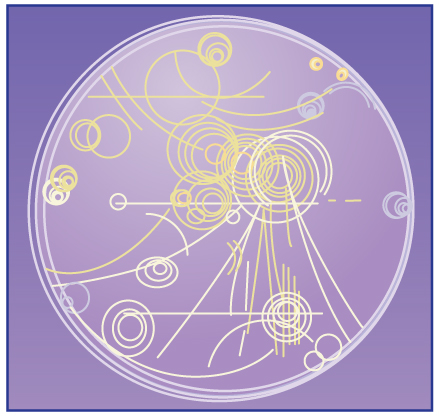Bubble Chamber: Trails of bubbles are produced by high-energy charged particles moving through the superheated liquid hydrogen in this artist’s rendition of a bubble chamber. There is a strong magnetic field perpendicular to the page that causes the curved paths of the particles. The radius of the path can be used to find the mass, charge, and energy of the particle.

So, does the magnetic force cause circular motion? Magnetic force is always perpendicular to velocity, so that it does no work on the charged particle. The particle’s kinetic energy and speed thus remain constant. The direction of motion is affected, but not the speed. This is typical of uniform circular motion. The simplest case occurs when a charged particle moves perpendicular to a uniform B-field, such as shown in. (If this takes place in a vacuum, the magnetic field is the dominant factor determining the motion. ) Here, the magnetic force (Lorentz force) supplies the centripetal force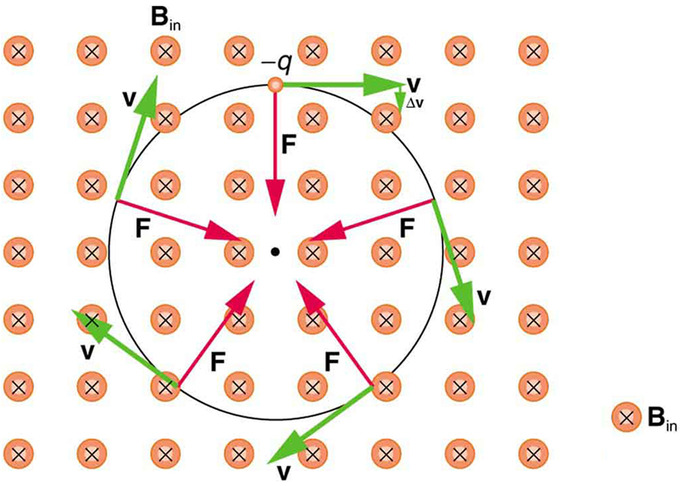Circular Motion of Charged Particle in Magnetic Field: A negatively charged particle moves in the plane of the page in a region where the magnetic field is perpendicular into the page (represented by the small circles with x’s—like the tails of arrows). The magnetic force is perpendicular to the velocity, and so velocity changes in direction but not magnitude. Uniform circular motion results.

$\text{F}_{\text{c}}=\frac{\text{mv}^{2}}{\text{r}}$.

Noting that

$\text{sin}\theta =1$

we see that

$\text{F}=\text{qvB}.$

The Lorentz magnetic force supplies the centripetal force, so these terms are equal:

$\text{qvB}=\frac{\text{mv}^{2}}{\text{r}}$

solving for r yields

$\text{r}=\frac{\text{mv}}{\text{qB}}$

Here, r, called the gyroradius or cyclotron radius, is the radius of curvature of the path of a charged particle with mass m and charge q, moving at a speed v perpendicular to a magnetic field of strength B. In other words, it is the radius of the circular motion of a charged particle in the presence of a uniform magnetic field. If the velocity is not perpendicular to the magnetic field, then v is the component of the velocity perpendicular to the field. The component of the velocity parallel to the field is unaffected, since the magnetic force is zero for motion parallel to the field. We’ll explore the consequences of this case in a later section on spiral motion.

A particle experiencing circular motion due to a uniform magnetic field is termed to be in a cyclotron resonance. The term comes from the name of a cyclic particle accelerator called a cyclotron, showed in. The cyclotron frequency (or, equivalently, gyrofrequency) is the number of cycles a particle completes around its circular circuit every second and can be found by solving for v above and substituting in the circulation frequency so that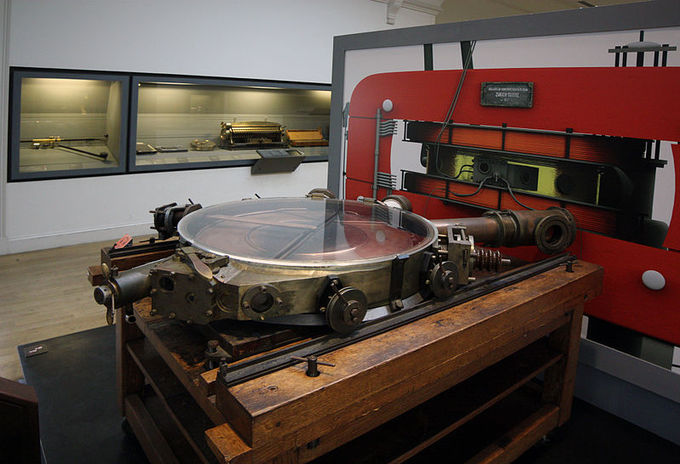Cyclotron: A French cyclotron, produced in Zurich, Switzerland in 1937

$\text{f}=\frac{\text{v}}{2 \pi \text{r}}$

becomes

$\text{f}=\frac{\text{qB}}{2 \pi \text{m}}$

The cyclotron frequency is trivially given in radians per second by

$\omega=\frac{\text{qB}}{\text{m}}$.

## Helical Motion

Helical motion results when the velocity vector is not perpendicular to the magnetic field vector.

### Learning Objectives

Describe conditions that lead to the helical motion of a charged particle in the magnetic field

### Key Takeaways

#### Key Points

• Previously, we have seen that circular motion results when the velocity of a charged particle is perpendicular to the magnetic field. The speed and kinetic energy of the particle remain constant, but the direction is altered at each instant by the perpendicular magnetic force.
• If the velocity is not perpendicular to the magnetic field, we consider only the component of v that is perpendicular to the field when making our calculations.
• The component of the velocity parallel to the field is unaffected, since the magnetic force is zero for motion parallel to the field. This produces helical motion.
• Charges may spiral along field lines. If the strength of the magnetic field increases in the direction of motion, the field will exert a force to slow the charges and even reverse their direction. This is known as a magnetic mirror.

#### Key Terms

• helical motion: The motion that is produced when one component of the velocity is constant in magnitude and direction (i.e., straight-line motion) while the other component is constant in speed but uniformly varies in direction (i.e., circular motion). It is the superposition of straight-line and circular motion.
• magnetic mirror: A magnetic field configuration where the field strength changes when moving along a field line. The mirror effect results in a tendency for charged particles to bounce back from the high field region.

### Helical Motion

In the section on circular motion we described the motion of a charged particle with the magnetic field vector aligned perpendicular to the velocity of the particle. In this case, the magnetic force is also perpendicular to the velocity (and the magnetic field vector, of course) at any given moment resulting in circular motion. The speed and kinetic energy of the particle remain constant, but the direction is altered at each instant by the perpendicular magnetic force. quickly reviews this situation in the case of a negatively charged particle in a magnetic field directed into the page.Circular Motion of Charged Particle in Magnetic Field: A negatively charged particle moves in the plane of the page in a region where the magnetic field is perpendicular into the page (represented by the small circles with x’s—like the tails of arrows). The magnetic force is perpendicular to the velocity, and so velocity changes in direction but not magnitude. Uniform circular motion results.

What if the velocity is not perpendicular to the magnetic field? Then we consider only the component of v that is perpendicular to the field when making our calculations, so that the equations of motion become:

$\text{F}_{\text{c}}=\frac{\text{mv}_{\perp}^2}{\text{r}}$

$\text{F}=\text{qvBsin} \theta=\text{qv}_{\perp}\text{B}$

The component of the velocity parallel to the field is unaffected, since the magnetic force is zero for motion parallel to the field. This produces helical motion (i.e., spiral motion) rather than a circular motion.

shows how electrons not moving perpendicular to magnetic field lines follow the field lines. The component of velocity parallel to the lines is unaffected, and so the charges spiral along the field lines. If field strength increases in the direction of motion, the field will exert a force to slow the charges (and even reverse their direction), forming a kind of magnetic mirror.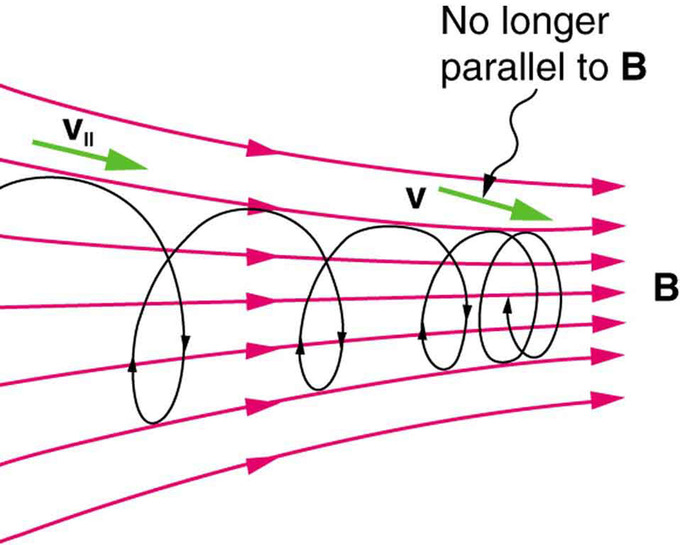Helical Motion and Magnetic Mirrors: When a charged particle moves along a magnetic field line into a region where the field becomes stronger, the particle experiences a force that reduces the component of velocity parallel to the field. This force slows the motion along the field line and here reverses it, forming a “magnetic mirror. “

The motion of charged particles in magnetic fields are related to such different things as the Aurora Borealis or Aurora Australis (northern and southern lights) and particle accelerators. Charged particles approaching magnetic field lines may get trapped in spiral orbits about the lines rather than crossing them, as seen above. Some cosmic rays, for example, follow the Earth’s magnetic field lines, entering the atmosphere near the magnetic poles and causing the southern or northern lights through their ionization of molecules in the atmosphere. Those particles that approach middle latitudes must cross magnetic field lines, and many are prevented from penetrating the atmosphere. Cosmic rays are a component of background radiation; consequently, they give a higher radiation dose at the poles than at the equator.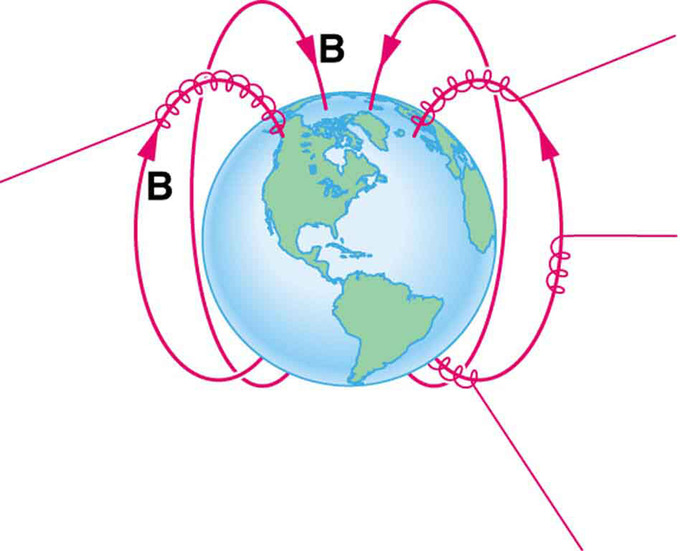Charged Particles Spiral Along Earth’s Magnetic Field Lines: Energetic electrons and protons, components of cosmic rays, from the Sun and deep outer space often follow the Earth’s magnetic field lines rather than cross them. (Recall that the Earth’s north magnetic pole is really a south pole in terms of a bar magnet. )

## Examples and Applications

Cyclotrons, magnetrons, and mass spectrometers represent practical technological applications of electromagnetic fields.

### Learning Objectives

Discuss application of mass spectrometers, movement of charged particles in a cyclotron, and how microwaves are generated in the cavity magnetron

### Key Takeaways

#### Key Points

• A cyclotron is a type of particle accelerator in which charged particles accelerate outwards from the center along a spiral path. The particles are held to a spiral trajectory by a static magnetic field and accelerated by a rapidly varying electric field.
• The cavity magnetron is a high-powered vacuum tube that generates microwaves using the interaction of a stream of electrons with a magnetic field. The magnetron has applications in radar, heating, and lighting.
• Mass spectrometers measure the mass-to-charge ratio of charged particles through the use of electromagnetic fields to segregate particles with different masses and/or charges. It can be used to determine the elemental composition of a molecule or sample.

#### Key Terms

• cyclotron: An early particle accelerator in which charged particles were generated at a central source and accelerated spirally outward through a fixed magnetic and alternating electric fields.
• mass spectrometer: A device used in mass spectrometry to discover the mass composition of a given substance.
• magnetron: A device in which electrons are made to resonate in a specially shaped chamber and thus produce microwave radiation; used in radar, and in microwave ovens.

### Overview

Recall that the charged particles in a magnetic field will follow a circular or spiral path depending on the alignment of their velocity vector with the magnetic field vector. The consequences of such motion can have profoundly practical applications. Many technologies are based on the motion of charged particles in electromagnetic fields. We will explore some of these, including the cyclotron and synchrotron, cavity magnetron, and mass spectrometer.

### Cyclotrons and Synchrotrons

A cyclotron is a type of particle accelerator in which charged particles accelerate outwards from the center along a spiral path. The particles are held to a spiral trajectory by a static magnetic field and accelerated by a rapidly varying (radio frequency ) electric field.Cyclotron Sketch: Sketch of a particle being accelerated in a cyclotron, and being ejected through a beamline.

Cyclotrons accelerate charged particle beams using a high frequency alternating voltage which is applied between two “D”-shaped electrodes (also called “dees”). An additional static magnetic field is applied in perpendicular direction to the electrode plane, enabling particles to re-encounter the accelerating voltage many times at the same phase. To achieve this, the voltage frequency must match the particle’s cyclotron resonance frequency,

$\text{f}=\frac{\text{qB}}{2 \pi \text{m}}$

with the relativistic mass m and its charge q. This frequency is given by equality of centripetal force and magnetic Lorentz force. The particles, injected near the center of the magnetic field, increase their kinetic energy only when recirculating through the gap between the electrodes; thus they travel outwards along a spiral path. Their radius will increase until the particles hit a target at the perimeter of the vacuum chamber, or leave the cyclotron using a beam tube, enabling their use. The particles accelerated by the cyclotron can be used in particle therapy to treat some types of cancer. Additionally, cyclotrons are a good source of high-energy beams for nuclear physics experiments.

A synchrotron is an improvement upon the cyclotron in which the guiding magnetic field (bending the particles into a closed path) is time-dependent, being synchronized to a particle beam of increasing kinetic energy. The synchrotron is one of the first accelerator concepts that enable the construction of large-scale facilities, since bending, beam focusing and acceleration can be separated into different components.

### Cavity Magnetron

The cavity magnetron is a high-powered vacuum tube that generates microwaves using the interaction of a stream of electrons with a magnetic field. All cavity magnetrons consist of a hot cathode with a high (continuous or pulsed) negative potential created by a high-voltage, direct-current power supply. The cathode is built into the center of an evacuated, lobed, circular chamber. A magnetic field parallel to the filament is imposed by a permanent magnet. The magnetic field causes the electrons, attracted to the (relatively) positive outer part of the chamber, to spiral outward in a circular path, a consequence of the Lorentz force. Spaced around the rim of the chamber are cylindrical cavities. The cavities are open along their length and connect the common cavity space. As electrons sweep past these openings, they induce a resonant, high-frequency radio field in the cavity, which in turn causes the electrons to bunch into groups.Cavity Magnetron Diagram: A cross-sectional diagram of a resonant cavity magnetron. Magnetic lines of force are parallel to the geometric axis of this structure.

The sizes of the cavities determine the resonant frequency, and thereby the frequency of emitted microwaves. The magnetron is a self-oscillating device requiring no external elements other than a power supply. The magnetron has practical applications in radar, heating (as the primary component of a microwave oven), and lighting.

### Mass Spectrometry

Mass spectrometry is an analytical technique that measures the mass-to-charge ratio of charged particles. It is used for determining masses of particles and determining the elemental composition of a sample or molecule.

Mass analyzers separate the ions according to their mass-to-charge ratio. The following two laws govern the dynamics of charged particles in electric and magnetic fields in a vacuum:

$\text{F}=\text{Q}(\text{E}+\text{v} \times \text{B})$ (Lorentz force)

$\text{F}=\text{ma}$

Equating the above expressions for the force applied to the ion yields:

$(\text{m}/\text{Q})\text{a}=\text{E}+\text{v} \times \text{B}$

This differential equation along with initial conditions completely determines the motion of a charged particle in terms of m/Q. There are many types of mass analyzers, using either static or dynamic fields, and magnetic or electric fields, but all operate according to the above differential equation.

The following figure illustrates one type of mass spectrometer. The deflections of the particles are dependent on the mass-to-charge ratio. In the case of isotopic carbon dioxide, each molecule has the same charge, but different masses. The mass spectrometer will segregate the particles spatially allowing a detector to measure the mass-to-charge ratio of each particle. Since the charge is known, the absolute mass can be determined trivially. The relative abundances can be inferred from counting the number of particles of each given mass.Mass Spectrometry: Schematics of a simple mass spectrometer with sector type mass analyzer. This one is for the measurement of carbon dioxide isotope ratios (IRMS) as in the carbon-13 urea breath test.